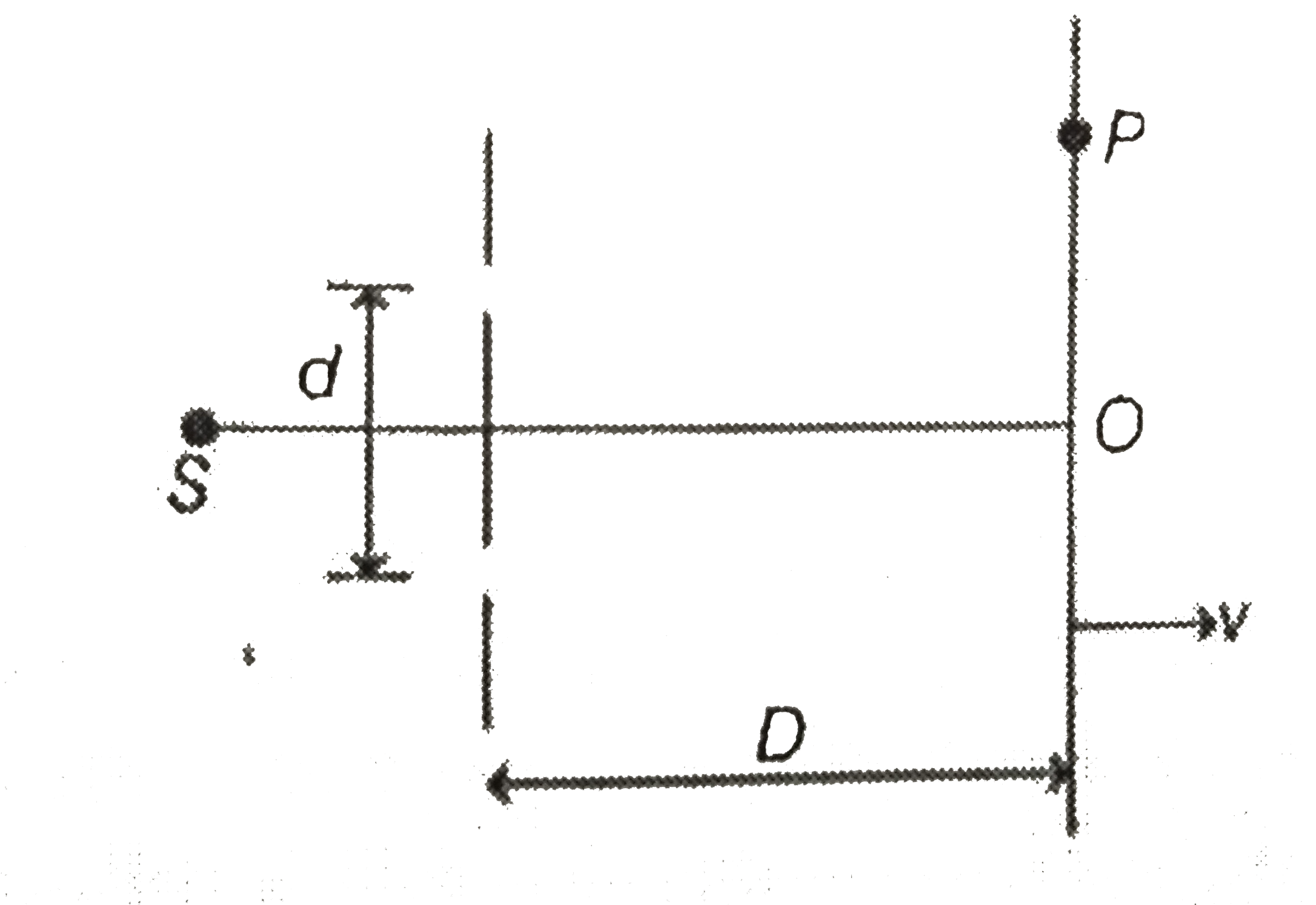# In YDSE apparatus shown in figure wavlength of light used is lambda. The screen is moved away form the source with a constant speed v. Initial dista

23 views
in Physics
In YDSE apparatus shown in figure wavlength of light used is lambda. The screen is moved away form the source with a constant speed v. Initial distance between screen and plane of slits was D.At a point P on the screen the order of fringe will
A. (2D)/(v)
B. (D)/(v)
C. (3D)/(2v)
D. (3D)/(v)

by (73.0k points)